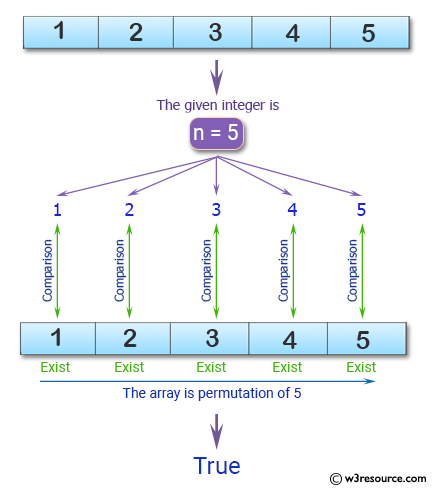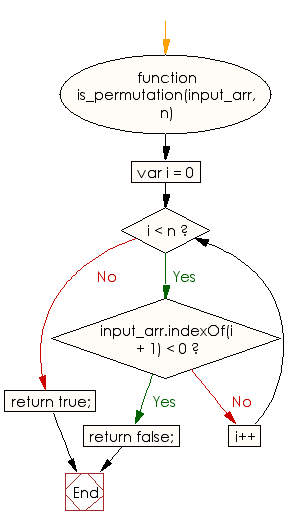# JavaScript: Find whether the members of a given array of integers is a permutation of numbers from 1 to a given integer

## JavaScript Basic: Exercise-123 with Solution

Write a JavaScript program to find whether the members of a given array of integers is a permutation of numbers from 1 to a given integer.

Pictorial Presentation:Sample Solution:

HTML Code:

``````<!DOCTYPE html>
<html>
<meta charset="utf-8">
<meta name="viewport" content="width=device-width">
<title> Find whether the members of a given array of integers is a permutation of numbers from 1 to a given integer</title>
<body>

</body>
</html>
```
```

JavaScript Code:

``````function is_permutation(input_arr, n) {
for (var i = 0; i < n; i++) {
if (input_arr.indexOf(i + 1) < 0)
return false;
}
return true;
}
console.log(is_permutation([1, 2, 3, 4, 5], 5));
console.log(is_permutation([1, 2, 3, 5], 5));
``````

Sample Output:

```true
false
```

Flowchart:ES6 Version:

``````function is_permutation(input_arr, n) {
for (let i = 0; i < n; i++) {
if (!input_arr.includes(i + 1))
return false;
}
return true;
}
console.log(is_permutation([1, 2, 3, 4, 5], 5));
console.log(is_permutation([1, 2, 3, 5], 5));
``````

Live Demo:

See the Pen javascript-basic-exercise-123 by w3resource (@w3resource) on CodePen.

Improve this sample solution and post your code through Disqus

What is the difficulty level of this exercise?

Test your Programming skills with w3resource's quiz.

﻿

## JavaScript: Tips of the Day

Returns the sum of the powers of all the numbers from start to end (both inclusive)

Example:

```const sumPower = (end, power = 2, start = 1) =>
Array(end + 1 - start)
.fill(0)
.map((x, i) => (i + start) ** power)
.reduce((a, b) => a + b, 0);
console.log(sumPower(10)); // 385
console.log(sumPower(10, 3)); // 3025
console.log(sumPower(10, 3, 5)); // 2925
```

Output:

```385
3025
2925
```